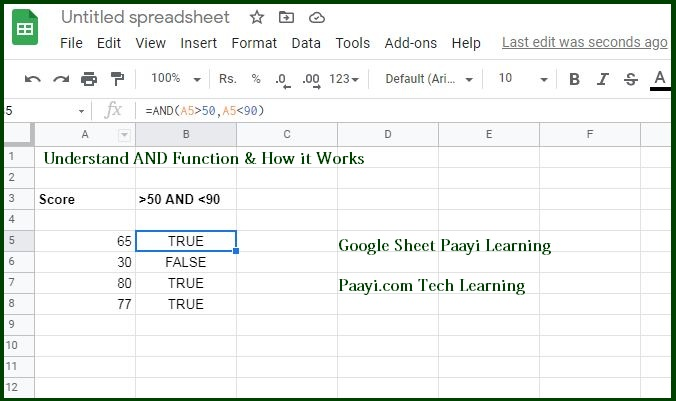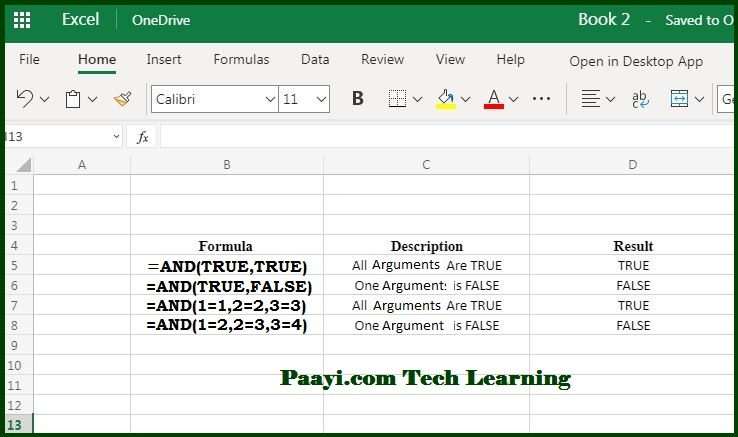# Learn How to Use Microsoft Excel AND Function

Written by | 0 Comments | 683 Views

In this article, you will learn how to use the Microsoft Excel AND function and its prime function in Microsoft Excel. You will also get to know the Microsoft Excel AND function return value and syntax with the help of some examples.

### How to Use AND Function in Microsoft Excel?

The AND function in Microsoft Excel is a logical function that is used to require more than one condition at the same time. Usually, the  AND function returns either TRUE or FALSE. In simple words, it is used in the logical functions to determine if all the conditions in the test are TRUE or FALSE. The AND function can be used in various ways to assess the logical conditions.### AND Function Syntax

=AND (logical1, [logical2],..)

The purpose of AND function is to test multiple conditions.

Arguments

• logical1 - It is the first condition or logical value to evaluate.
• logical2 - It is the second condition or logical value to assess.

### Examples of AND Functions Usages

Example 1. This example will help you understand the primary usage of AND function.

Example 2. This example will help you understand the AND function more appropriately.Usage of AND Function

• The AND function is utilized to check more than one logical value at the same time, up to 255 conditions.
• The AND function evaluates all the values provided and returns TRUE if the value evaluated is TRUE.
• The AND function evaluates all the values provided and returns FALSE if the value evaluated is FALSE.
• AND function like the OR function aggregate results to a single value. That means both of them can't be used in the array operations.

Essential Features of AND Function

• The AND function is not case-sensitive.
• The AND function does not support wildcards. The wildcards are the special characters that let you perform some fuzzy operation in Microsoft Excel.
• Text values or empty cells/arguments provided will be ignored.
• The AND function will return #VALUE if no logical values are found during the evaluation.